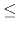# Non-negative Partial Sums

You are given a sequence of n numbers a0,..., an-1. A cyclic shift by k positions ( 0kn - 1) results in the following sequence: ak, ak+1,..., an-1, a0, a1,..., ak-1. How many of the n cyclic shifts satisfy the condition that the sum of the first i numbers is greater than or equal to zero for all i with 1in?

## Input

Each test case consists of two lines. The first contains the number n ( 1n106), the number of integers in the sequence. The second contains n integers a0,..., an-1 ( -1000ai1000) representing the sequence of numbers. The input will finish with a line containing 0.

## Output

For each test case, print one line with the number of cyclic shifts of the given sequence which satisfy the condition stated above.

```3
2 2 1
3
-1 1 1
1
-1
0
```

```3
2
0
```